# Point

## 说明

``````point = arcpy.Point(25282, 43770)
ptGeometry = arcpy.PointGeometry(point)``````

## 语法

` Point ({X}, {Y}, {Z}, {M}, {ID})`
 参数 说明 数据类型 X 点的 X 坐标。(默认值为 0.0) Double Y 点的 Y 坐标。(默认值为 0.0) Double Z 点的 Z 坐标。(默认值为 None) Double M 点的 M 值。(默认值为 None) Double ID 点的形状 ID。(默认值为 0) Integer

## 属性

 属性 说明 数据类型 ID(可读写) 用于唯一标识点的整数。 Integer M(可读写) 点的测量值。 Double X(可读写) 点的水平坐标。 Double Y(可读写) 点的垂直坐标。 Double Z(可读写) 点的高程值。 Double

## 方法概述

 方法 说明 clone (point_object) 克隆 Point 对象。 contains (second_geometry, {relation}) 指明基础几何中是否包含比较几何。contains 与 within 相反。本图仅显示 True 关系。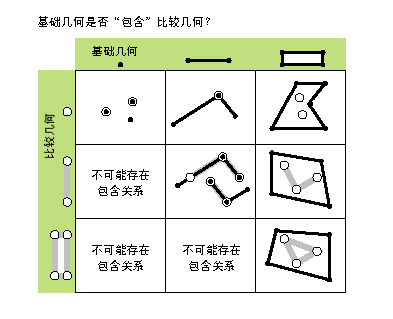crosses (second_geometry) 指明两个几何是否相交于较小形状类型的几何。 如果两条折线仅共用公共点（至少有一个点不是端点），则这两条折线交叉。如果折线和面在面（不等于整条折线）的内部共享一条折线或一个公共点（对于垂线），那么该折线与面交叉。本图仅显示 True 关系。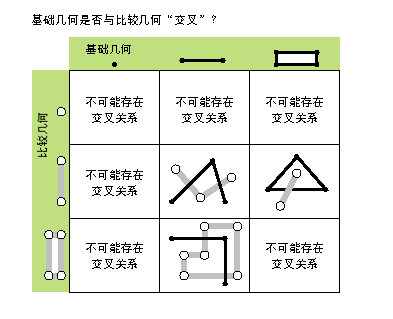disjoint (second_geometry) 指明基础几何和比较几何是否未共用任何点。如果 disjoint 返回 False，则两个几何相交。本图仅显示 True 关系。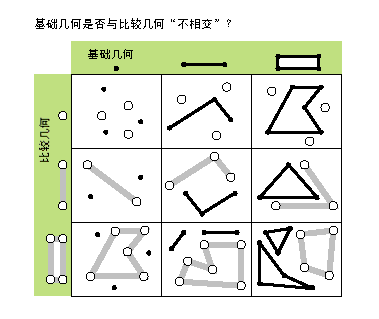equals (second_geometry) 指示原几何和参照几何的 shape 类型是否相同并在平面中定义相同点集。这仅是 2D 的比较；已忽略 M 值和 Z 值。本图仅显示 True 关系。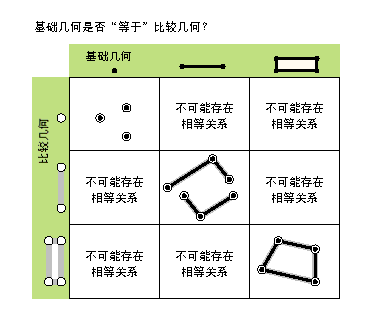overlaps (second_geometry) 指示两个几何的交集是否具有与其中一个输入几何相同的形状类型，并且不等于任一输入几何。 本图仅显示 True 关系。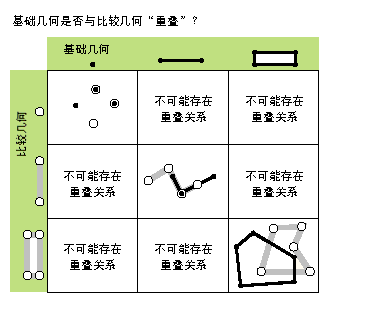touches (second_geometry) 指示几何的边界是否相交。 当两个几何的交集不为空，但它们内部的交集为空时，说明两个几何接触。例如，仅当点与折线的一个终点重合时，才表示点与折线接触。本图仅显示 True 关系。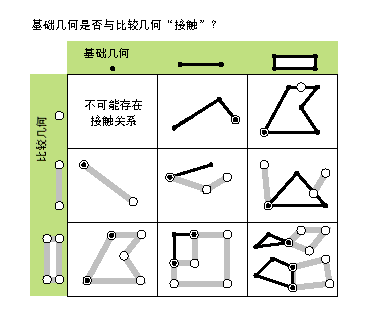within (second_geometry, {relation}) 指明基础几何是否位于比较几何之内。within 与运算符 contains 相反。本图仅显示 True 关系。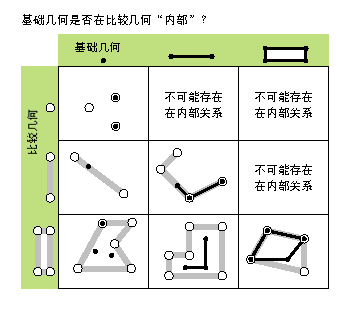如果基础几何是这些几何的交集且其内部交集不为空，则基础几何位于比较几何之内。within 是 Clementini 运算符，空的基础几何除外。

## 方法

`clone (point_object)`
 参数 说明 数据类型 point_object A Point object. Point
`contains (second_geometry, {relation})`
 参数 说明 数据类型 second_geometry 第二个几何。 Object relation 空间关系类型。BOUNDARY— 关系对内部或边界没有任何限制。CLEMENTINI— 几何内部必须相交。指定 CLEMENTINI 等于指定 None。这是默认设置。PROPER— 几何边界不得相交。(默认值为 None) String

 数据类型 说明 Boolean 返回的布尔值 True 表示该几何包含第二个几何。
`crosses (second_geometry)`
 参数 说明 数据类型 second_geometry 第二个几何。 Object

 数据类型 说明 Boolean 返回布尔值为 True 指明两个几何相交于较小形状类型的几何。
`disjoint (second_geometry)`
 参数 说明 数据类型 second_geometry 第二个几何。 Object

 数据类型 说明 Boolean 返回布尔值 True 表示两个几何未共用任何点。
`equals (second_geometry)`
 参数 说明 数据类型 second_geometry 第二个几何。 Object

 数据类型 说明 Boolean 返回布尔值为 True 表示两个几何的 shape 类型相同并在平面中定义了相同点集。
`overlaps (second_geometry)`
 参数 说明 数据类型 second_geometry 第二个几何。 Object

 数据类型 说明 Boolean 返回布尔值为 True 表示两个几何的交集具有与其中一个输入几何相同的尺寸。
`touches (second_geometry)`
 参数 说明 数据类型 second_geometry 第二个几何。 Object

 数据类型 说明 Boolean 返回布尔值为 True，表示几何的边界相交。
`within (second_geometry, {relation})`
 参数 说明 数据类型 second_geometry 第二个几何。 Object relation 空间关系类型。BOUNDARY— 关系对内部或边界没有任何限制。CLEMENTINI— 几何内部必须相交。指定 CLEMENTINI 等于指定 None。这是默认设置。PROPER— 几何边界不得相交。(默认值为 None) String

 数据类型 说明 Boolean 返回布尔值 True 表示该几何被包含在另一个几何之内。

## 代码示例

``````import arcpy

# Create point object
point = arcpy.Point(2000, 2500)

# Print point properties
print("Point properties:")
print(" ID: {0}".format(point.ID))
print(" X:  {0}".format(point.X))
print(" Y:  {0}".format(point.Y))``````

``````import arcpy

# Create cursor to retrieve Hawaii shape
feature_class = "c:/data/Hawaii.shp"
cursor = arcpy.da.SearchCursor(feature_class, ["SHAPE@"])

for row in cursor:
# Get the geometry object from the shape field
print("Number of Hawaiian islands: {0}".format(row.partCount))

# GetPart returns an array of point objects for each part.
for island in row.getPart():
print("Vertices in island: {0}".format(island.count))
for point in island:
print("X: {0}, Y: {1})".format(point.X, point.Y))``````